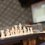# Select a number (Rules)

To play this game, look at the set Select A Number.

Based on Can you outsmart everyone and Greed Control, I've decided to create something similar with the following rules.

1. For each round, you can only comment one positive integer between the range given by replying under the "Submit your number here" comment.

2. One person can only comment at most 1 positive integer. State this explicitly.

3. Any person commenting more than 1 positive integer in the "Submit your number here" comment will be disqualified for the round.

4. Your score for the round will be (number picked) / (number of people choosing the number). (e.g., if you choose 20, and only 4 people in the round choose 20, then you get 20/4 = 5 points. However, if you choose 9 and no one else choose 9, you get 9 points.)

5. The round will be stopped after a random amount of time. (Range stated above every round.)

6. Any late entries will NOT be accepted.

7. To reward the first ones to submit, the first $n$ person to submit valid submissions will receive $a_1, a_2, ..., a_n$ points where $a_1, a_2, ..., a_n$ are positive real numbers and are stated every round. Also, $n$ will be stated every round.

8. Also, an extra random positive real amount of bonus points (whose range will be stated every round and actual value determined after closing.) will be awarded to the first $k$ submitters. ($k$ will also be stated each round.)

9. The points are rounded to 2 decimal places.

10. The game will end after 20 rounds.

11. The one with the most points after the 20 rounds wins the game.

12. You may not edit your entry.

Clarification for 8 : Every player who receive the random amount of bonus points will get the same score.Note by Zi Song Yeoh
6 years ago

This discussion board is a place to discuss our Daily Challenges and the math and science related to those challenges. Explanations are more than just a solution — they should explain the steps and thinking strategies that you used to obtain the solution. Comments should further the discussion of math and science.

When posting on Brilliant:

• Use the emojis to react to an explanation, whether you're congratulating a job well done , or just really confused .
• Ask specific questions about the challenge or the steps in somebody's explanation. Well-posed questions can add a lot to the discussion, but posting "I don't understand!" doesn't help anyone.
• Try to contribute something new to the discussion, whether it is an extension, generalization or other idea related to the challenge.

MarkdownAppears as
*italics* or _italics_ italics
**bold** or __bold__ bold
- bulleted- list
• bulleted
• list
1. numbered2. list
1. numbered
2. list
Note: you must add a full line of space before and after lists for them to show up correctly
paragraph 1paragraph 2

paragraph 1

paragraph 2

[example link](https://brilliant.org)example link
> This is a quote
This is a quote
    # I indented these lines
# 4 spaces, and now they show
# up as a code block.

print "hello world"
# I indented these lines
# 4 spaces, and now they show
# up as a code block.

print "hello world"
MathAppears as
Remember to wrap math in $$ ... $$ or $ ... $ to ensure proper formatting.
2 \times 3 $2 \times 3$
2^{34} $2^{34}$
a_{i-1} $a_{i-1}$
\frac{2}{3} $\frac{2}{3}$
\sqrt{2} $\sqrt{2}$
\sum_{i=1}^3 $\sum_{i=1}^3$
\sin \theta $\sin \theta$
\boxed{123} $\boxed{123}$

Sort by:

What's the start time? "Randomly" selected?

Staff - 6 years ago

41

- 6 years ago

- 6 years ago

Oh, this is only the rules section, there's another note in the set, please check it out.

- 6 years ago

Yeah, I realised later.

- 6 years ago

I added a link to the set, so that it's more obvious.

Interesting game.

Staff - 6 years ago